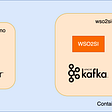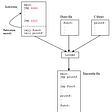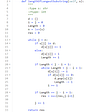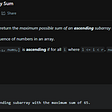# 0/1 Knapsack Problem(Recursive) — Day 41(Python)

Today, I will not be solving a leetcode problem. I always had trouble understanding Dynamic Programming and Recursions. I try to look for good Youtubers who can explain to me Dynamic Programming. My brother suggested me to watch Aditya Verma’s youtube channel. I loved the way he explained the algorithm and intuitions that run behind the solutions. So today, I thought I would try solving the Knapsack problem the way Aditya Verma explains in his videos. I will add the link to his video at the end of my article.

Dynamic Programming is a method to solve a given problem by breaking it down into simpler sub-problems, which means you can solve the given using recursion too. Dynamic programming is an optimization over recursion. While using recursion, there is a possibility of repeating the calculations. Through dynamic programming, you save the intermediate results to avoid recalculation.

Let us look at what repeating calculations mean. In the below image, we see how we calculate the 5th Fibonacci term through recursion. The circles in red are the repeated calculation. We can see that the 3rd Fibonacci term is called twice while the 2nd Fibonacci term is called thrice. To avoid unnecessary calculations, we make use of dynamic programming. We can store the result of Fibonacci 3 and Fibonacci 2 in a dictionary, such that whenever we are required, we can get the values from the dictionary instead of recalculating.

To understand if a given problem can be solved via Dynamic Programming, it has to satisfy the following two conditions.

1. You will always have some set of choices. E.g. should you be including an item in the knapsack, should you include coins, etc
2. The problem would require you to find an optimal value, say find maximum, find the minimum, find largest, etc.

0–1 Knapsack Problem

You are given weights and values of N items, put these items in a knapsack of capacity W to get the maximum total value in the knapsack. Note that we have only one quantity of each item.
In other words, given two integer arrays, val[0..N-1] and wt[0..N-1] represent values and weights associated with N items respectively. Also given an integer W which represents knapsack capacity, find out the maximum value subset of val[] such that sum of the weights of this subset is smaller than or equal to W. You cannot break an item, either pick the complete item or don’t pick it (0–1 property).

Example 1:

`Input:N = 3W = 4values[] = {1,2,3}weight[] = {4,5,1}Output: 3`

Example 2:

`Input:N = 3W = 3values[] = {1,2,3}weight[] = {4,5,6}Output: 0`

Complete the function knapSack() which takes maximum capacity W, weight array wt[], value array val[], and the number of items n as a parameter and returns the maximum possible value you can get.

Expected Time Complexity: O(N*W).
Expected Auxiliary Space: O(N*W)

Constraints:
1 ≤ N ≤ 1000
1 ≤ W ≤ 1000
1 ≤ wt[i] ≤ 1000
1 ≤ v[i] ≤ 1000

Let us understand the question. Consider yourself to be a thief. You decide to rob a house. You have a Knapsack in which you will put the items that you want to steal. But the Knapsack can only hold items until a certain weight.

Now, when you decide to steal, you would want to maximize the profit that you can get. You want to find a combination of items, such that the total weight of the items that you decide to steal is less than or equal to the total weight the knapsack can hold, and the profit you earn out of it is maximum.

The given problem is divided into smaller sub-problems, where we take each item and check if we should include it into our knapsack. The below diagram gives us conditions whether we should include the item in our knapsack or not. “W” represents the total weight the knapsack can hold. “w1” represents the weight of a single item. If the weight of the item is smaller than or equal to the weight of the knapsack, we have two choices; either to include the item in the knapsack or to avoid it.

Since we are thinking of a recursive solution, we should know our base condition. In this case, we should stop when there is no item in our list of items i.e. n is equal to 0 or the weight of the knapsack is equal to 0. Next, we need to make choices; if we decide to include the item in our knapsack we should reduce the weight of the item from the total weight knapsack can hold. Regardless of whether we include our item in the knapsack, we should remove that item from our list while making the next recursive call, hence we should decrement the value of “n”.

Let us look into the code snippet

`def knapSack(W, wt, val, n):    '''    :param W: capacity of knapsack     :param wt: list containing weights    :param val: list containing corresponding values    :param n: size of lists    :return: Integer    '''    # code here    if n == 0 or W == 0:        return 0    if wt[n-1] <= W:        return (max(val[n-1]+knapSack(W-wt[n-1], wt, val, n-1), knapSack(W, wt, val, n-1)))    else:        return (knapSack(W, wt, val, n-1))`

Complexity analysis.

Time Complexity

We have N items and each time we have 2 choices, whether to include the item or to discard it. Hence the time complexity is O(2^N).

Space Complexity.

We are making use of recursive calls that internally hold the stack, hence the space complexity is O(2^N).

I will be writing the second part of this problem which includes the Memoization approach to solve this problem.

I would love to hear your feedback about my posts. Do let me know if you have any comments or feedback.

--

--

--

## More from Annamariya Tharayil

Software Engineer. Find me @ www.linkedin.com/in/annamariya-jt

Love podcasts or audiobooks? Learn on the go with our new app.

## 9 Steps to Connect Database to Laravel## Creating a reactive GraphQL server with Spring Boot and Kotlin## BatchConn — sendmmsg/recvmmsg in Go## WSO2 Streaming Integrator Demos Using Pre-baked Docker Images## What I’ve learned about real life from programing## Dynamic Firewalling with Palo Alto Networks NGFWs and Consul-Terraform-Sync## Static and Dynamic library in C simplified.## Introducing Vuilder DAO## Annamariya Tharayil

Software Engineer. Find me @ www.linkedin.com/in/annamariya-jt

## LeetCode Must do Coding Problems Collection## CS50 Week5 Data Structure## LeetCode 1800. Maximum Ascending Subarray — Python Solution## Recursion. Recursio. Recursi. Recurs… What is recursion?